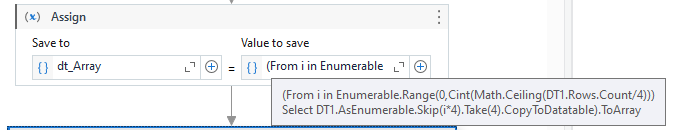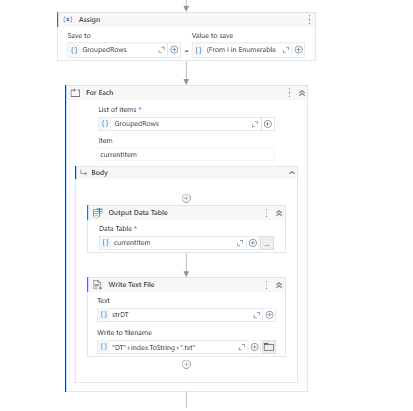# Split excel record

I have one excel sheet contains 8 records in it. I needs to write all those records in text file. For writing in text file there is one condition. Condition is you can write only 4 records in one excel file. next 4 records in excel should write in different text file .for each (set of 4) next records this process is repeat. How can i do this?
@Anil_G @Sudharsan_Ka @supermanPunch @Gokul001

• tried this linq query Dim rowsGrouped = From row In dtExcel.AsEnumerable()
Group row By GroupNumber = (dtExcel.Rows.IndexOf(row) \ 4) Into Group
Select Group.CopyToDataTable()
but it wont works

You could try to use the Skip and Take methods of the Datatable and prepate the array of 4 Records datatable.

Next, we can loop through these array of datatables one by one using a `For Each` activity and use `Output Datatable` Activity to convert the Datatable to a String, then write this string value to a text file using `Write Text File` activity.

For Preparing the 4 row Datatables, check the below :

``````dt_Array = (From i in Enumerable.Range(0,Cint(Math.Ceiling(DT.Rows.Count/4))) Select DT.AsEnumerable.Skip(i*4).Take(4).CopyToDatatable).ToArray
``````

Here, `dt_Array` is a variable of type Array of Datatable, `DT` is the datatable that contains the data from Excel sheet.

Let us know if you were able to implement this.

in for each loop give Enumerable.Range(0,Cint(Math.Ceiling(dtExcel.RowCount/4))).ToArray

try dtExcel.asenumerable().Skip(iterationnumber*4).Take(4).copytodatatable

next write to text file

Regards

Is the `.ToArray` added at the end of the expression ?

yes it is …

@pravin_bindage

1. Use for loop with `Enumerable.Range(0,Math.Ceil(dt.RowCount/4)).ToArray` and change type argument to integer
2. Inside loop use output datatable and give datatable as `dt.AsEnumerable.Skip(currentitem*4).Take(4).CopyToDataTable`
3. USe the output to write to textfile and textfile name can be `"Text" +currentitem.ToString + ".txt"`

cheers

It does work for me. Try to remove the Expression and add it again and check.Can please elaborate more? I didnt get it

It saprates datatable but it wont enters in loop

@pravin_bindage

I am looping through the enumerable.range which creates numers from 0 to datatable count divided by 4…as you need 4 record
Inside loop getting the required 4 rows for each iteration
then changing it to string and writing to text file

cheers

Do Check the steps mentioned, we do not need to use a `For Each Row` activity if the intention is to get the Datatable of 4 records into a separate text file.

We only need the First `For Each` activity that you have used, then use the `Output Datatable` actvity, this will convert the datatable to string. Use this String value and write it to a Text File with a dynamic name which we can get it from `For Each` Index property.

Something in the below way :Here, `index` is created in the index property of For Each.

Can you try with these steps-

1. Read the Excel sheet using the “Read Range” activity to get all the records into a datatable.
2. Initialize a counter variable to keep track of the number of records written.
3. Loop through each row in the datatable using a “For Each Row” activity.
4. Inside the loop, use an “If” activity to check if the counter is less than or equal to 4. If it is, then write the record to a text file using the “Append Line” activity, and increment the counter by 1.
5. If the counter becomes greater than 4, then close the current text file and reset the counter to 1. Open a new text file for writing using the “Create Text File” activity, and write the current record to the new text file.
6. Continue this process for all the records in the Excel sheet.
1 Like

This topic was automatically closed 3 days after the last reply. New replies are no longer allowed.